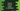# Python program to convert inches to millimeters## Python program to convert inches to millimeters:

In this post, we will learn how to convert inch to millimeter in Python. This program will take the inch value as the input from the user, convert it to millimeter and print it out.

## Inches to millimeter conversion formula:

1 inch is equal to 25.4 millimeter. So, if we multiply the inch value by 25.4, it will give us the millimeter value.

``1 inch = 25.4 millimeter``
``x inch = x * 25.4 millimeter``

x can be any number.

### Algorithm for the program:

This program will use the below algorithm:

• Take the inches as input from the user.
• Convert this value to millimeter by using the above formula.
• Print out the millimeter value.

### Python program to convert inch to millimeter:

Let’s write down the program using this algorithm:

``````inch = float(input("Enter the inch value: "))
milli = inch * 25.4

print("Millimeter: ", milli)``````

If you run this program, it will print output as like below:

``````Enter the inch value: 5.24
Millimeter:  133.096``````

### Reformating the result value up to 2 decimal places:

We can format the output to 2 decimal places by using the round method. The second parameter of this method should be 2 for that.

``````inch = float(input("Enter the inch value: "))
milli = round(inch * 25.4, 2)

print("Millimeter: ", milli)``````

It will give output like below:

``````Enter the inch value: 5.24
Millimeter:  133.1``````